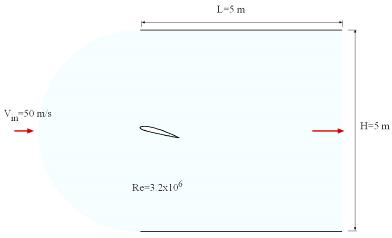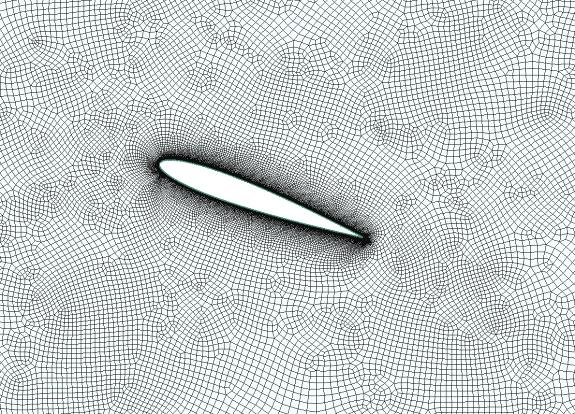Laminar unsteady flow around a cylinder
 Natural convection inside a cylindrical annulus (laminar, steady state)
 · Turbulent flow around a NACA 2412 airfoil—unstructured mesh
 · Natural convection inside a cylindrical annulus (laminar, steady state) ·
 ExamplesEasyCFD
 Numerical Calculation of Fluid Flow and Heat Transfer in 2D and 3D axisymmetric domains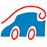· Pulsatile transient flow inside a channel transfer ·Detail of the grid generated around the cylinder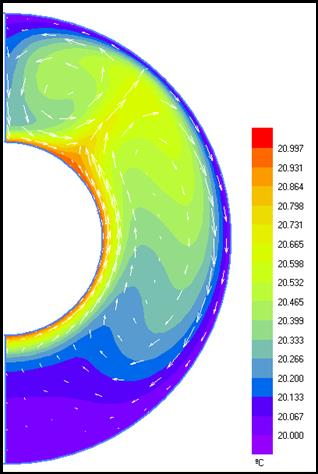Temperature contours and velocity vectorial field. Second solution from bifurcation, showing two vortices (c.f. Tutorial video).Problem definition:
 Problem definition for time instant = 0s :
 Problem definition :
 Temperature contours along and velocity vectorial field. Time instant = 6.0 s.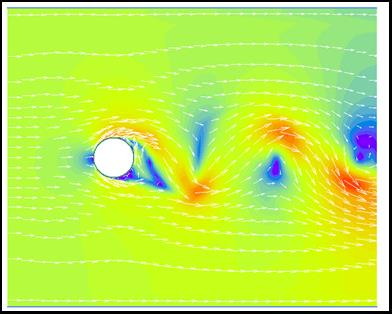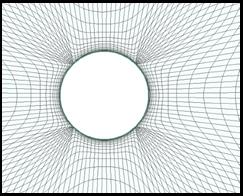Vortex shedding
 · Turbulent flow around a car shape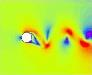Tutorial Movies:Tutorial Movies:Tutorial Movies: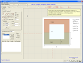Structured generalised mesh over a vehicle
 Streamlines and velocity contours for the flow over a vehicle
 Turbulent flow over a car shapeBelow you´ll find some examples of EasyCFD calculations. The provided tutorial movies are the best way to learn how to work with EasyCFD. They show you all the steps for the problem definition, calculation and post-processing for theses examples. Please consider meshes used in these examples and the surrounding domain dimensions (cylinder and Naca cases) are not optimized.   Visualization movies and tutorial movies are encoded in XviD MPEG-4 Codec. If you are not able to see these movies, you can download the XviD MPEG-4 Codec here.
 This example concerns the laminar flow around a cylinder, at Re=3.7E3. The tutorial shows the steps for geometry construction, imposition of boundary conditions, solver and post. The visualization movie is an animation showing the development of the von Karman street (vortex shedding).
 This example illustrates the calculation of a laminar natural convection flow inside a concentric annulus, for Pr=0.7 and Gr=5.0E4. This problem is characterized by a bifurcation, corresponding to the existence of 2 solutions (documented in the literature). The tutorial shows how to obtain each of these solutions.
 This is a problem of conjugate heat transfer, where both solid conduction and fluid convection are solved simultaneously. A fluid core is surrounded by two blocks of different materials (copper and steel). At the initial time instant, the fluid is at uniform temperature of 20ºC, while the copper and the steel blocks are at 15ºC and 30ºC, respectively.  The outer boundaries are adiabatic. The problem is solved for the time evolution of this system.
 This is an example of a turbulent flow calculation flow around a vehicle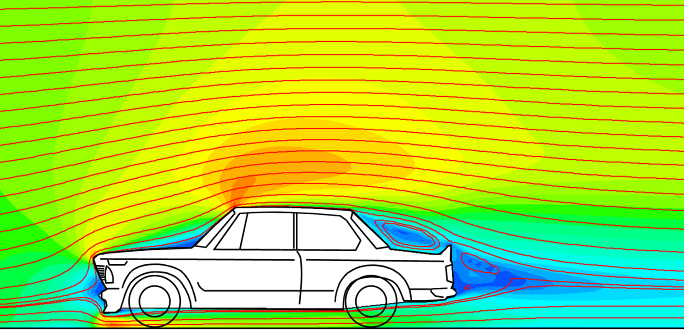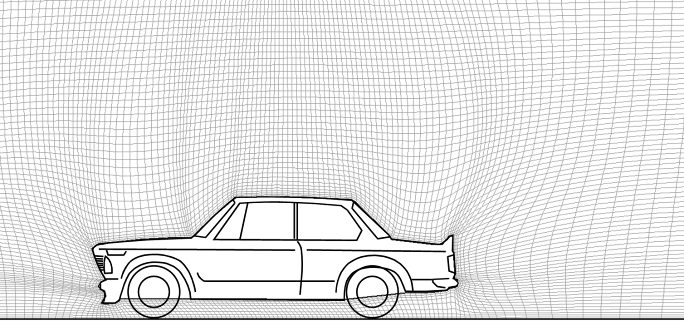Pulsatile Flow Inside a Symmetric Channel with Wavy WallsThis example illustrates the utilization of TableFunctions to assign boundary values. The inlet/outlet flow velocity is time dependent, corresponding to a cycle which includes flow reversal.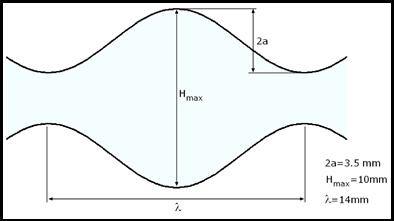Inlet Reynolds number as a function of time.
 Main geometrical parameter. Cf. EasyCFD user´s manual for the complete problem characterization.
 Visualization  Movie
 Visualization  MovieVisualization  Movie· Conjugate heat transfer (laminar, unsteady) ·U-Type Structured MeshC-Type Structured Mesh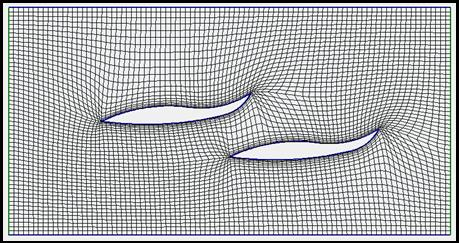Split  Structured MeshMeshing CapabilitiesUnstructured MeshTurbulent flow around a NACA 2412 airfloilLift coefficient versus incidence angle. Reynolds = 3.2E6.
 Problem definition.Tutorial Movie:This example illustrates the calculation of turbulent flow over a NACA 2412 airfoil, at Re=3.2E6, using an unstructured mesh. Besides the usual features, the tutorials show the geometry import from a text file containing the x and y coordinates of several points along the profile. From this initial geometry configuration, a rotation is then applied to the desired incidence angle.